# DESCRIPTIVE STATISTICS 1 DESCRIPTIVE STATISTICS Descriptive statistics are

• Slides: 32DESCRIPTIVE STATISTICS 1DESCRIPTIVE STATISTICS Descriptive statistics are summary measures which define some important characteristics of data. 1. Measures of location i. Measures of central tendency ii. Measures of position 2. Measures of dispersion (variation) 2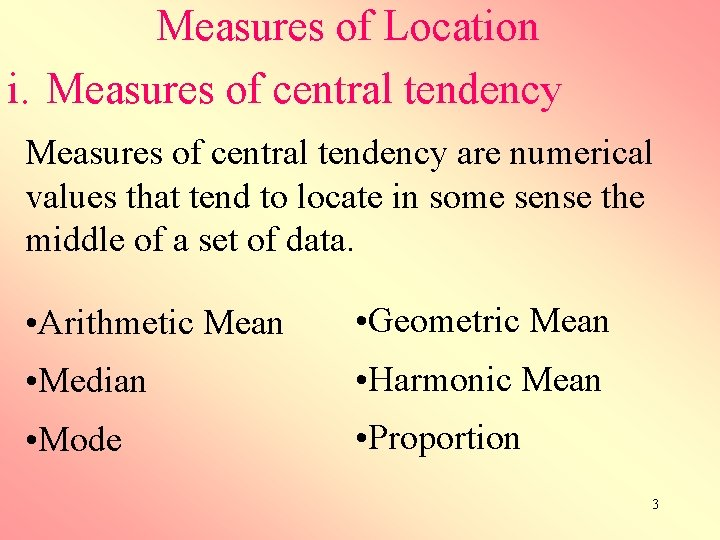Measures of Location i. Measures of central tendency are numerical values that tend to locate in some sense the middle of a set of data. • Arithmetic Mean • Geometric Mean • Median • Harmonic Mean • Mode • Proportion 3Measures of central tendency Arithmetic Mean (Mean) The arithmetic mean is the most common measure of the central tendency and is commonly used for symetrical distributions. It is used to summarize quantitative data. Sum of all the observations ( ) divided by the number of the observations (n). 4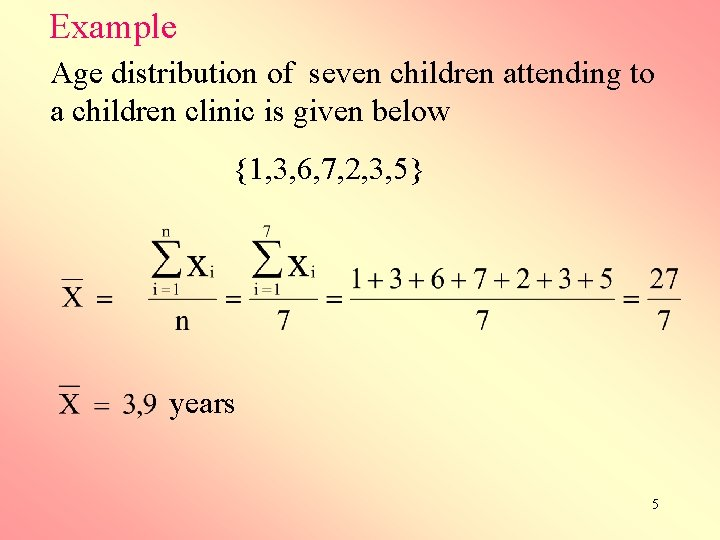Example Age distribution of seven children attending to a children clinic is given below {1, 3, 6, 7, 2, 3, 5} years 5Median The median is the middle value of the set of data when the data are ranked in order according to magnitude. When the data are put in order 50 % of the observations are less than or equal to the median, the rest is greater than the median. Median value is observation. 6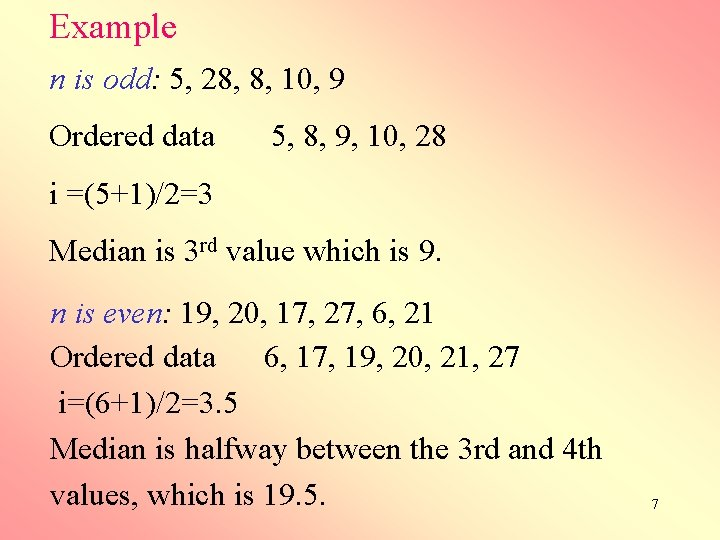Example n is odd: 5, 28, 8, 10, 9 Ordered data 5, 8, 9, 10, 28 i =(5+1)/2=3 Median is 3 rd value which is 9. n is even: 19, 20, 17, 27, 6, 21 Ordered data 6, 17, 19, 20, 21, 27 i=(6+1)/2=3. 5 Median is halfway between the 3 rd and 4 th values, which is 19. 5. 7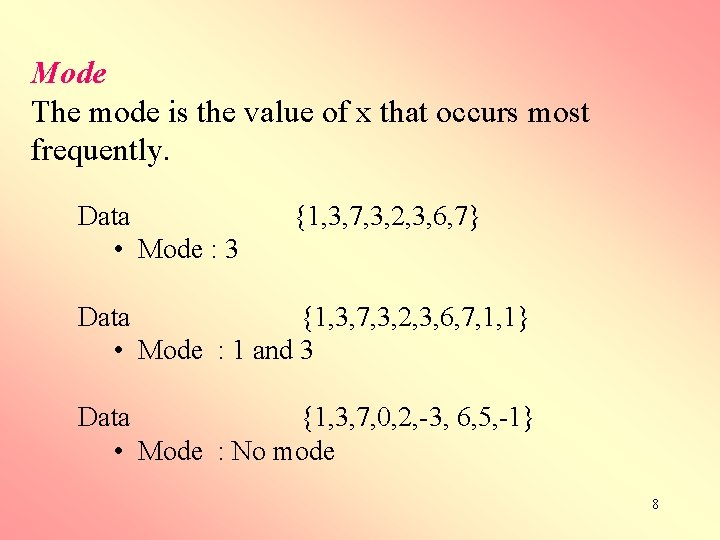Mode The mode is the value of x that occurs most frequently. Data • Mode : 3 {1, 3, 7, 3, 2, 3, 6, 7} Data {1, 3, 7, 3, 2, 3, 6, 7, 1, 1} • Mode : 1 and 3 Data {1, 3, 7, 0, 2, -3, 6, 5, -1} • Mode : No mode 8Example Suppose the age in years of the first 10 subjects enrolled in your study are: 34, 24, 56, 52, 21, 44, 64, 42, 46 Then the mean age of this group is 42. 7 years To find the median, first order the data: 21, 24, 34, 42, 44, 46, 52, 56, 64 The median is (44+44)/2 = 44 years The mode is 44 years. 9Suppose the next patient enrolls and her age is 97 years. How does the mean, median and mode change? Ordered data: 21, 24, 34, 42, 44, 46, 52, 56, 64, 97 Mean is 47, 6 42, 7 Median is 44 44 Mode is 44 44 10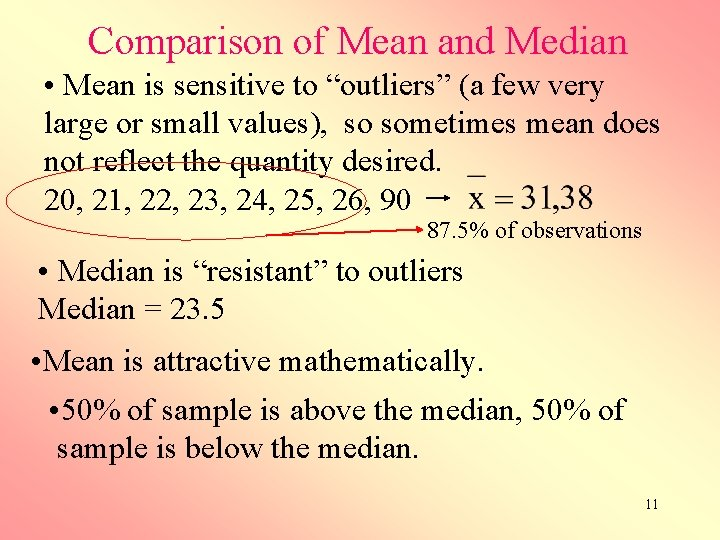Comparison of Mean and Median • Mean is sensitive to “outliers” (a few very large or small values), so sometimes mean does not reflect the quantity desired. 20, 21, 22, 23, 24, 25, 26, 90 87. 5% of observations • Median is “resistant” to outliers Median = 23. 5 • Mean is attractive mathematically. • 50% of sample is above the median, 50% of sample is below the median. 11Geometric mean is a summary statistic useful when the measurement scale is not linear, it is computed as or For example, in the area of psychometrics it is well known that the rated intensity of a stimulus (e. g. , brightness of a light) is often a logarithmic function of the actual intensity of the stimulus (brightness measured in units of Lux). In this instance, the geometric mean is a better "summary" of ratings than the simple mean. 12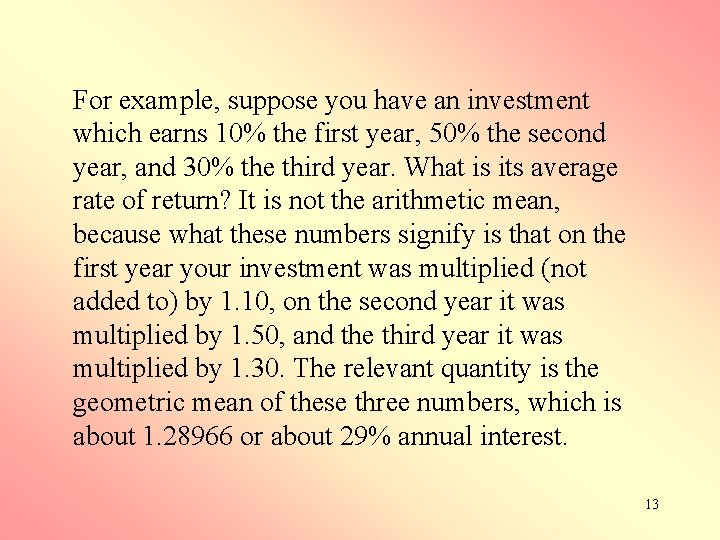For example, suppose you have an investment which earns 10% the first year, 50% the second year, and 30% the third year. What is its average rate of return? It is not the arithmetic mean, because what these numbers signify is that on the first year your investment was multiplied (not added to) by 1. 10, on the second year it was multiplied by 1. 50, and the third year it was multiplied by 1. 30. The relevant quantity is the geometric mean of these three numbers, which is about 1. 28966 or about 29% annual interest. 13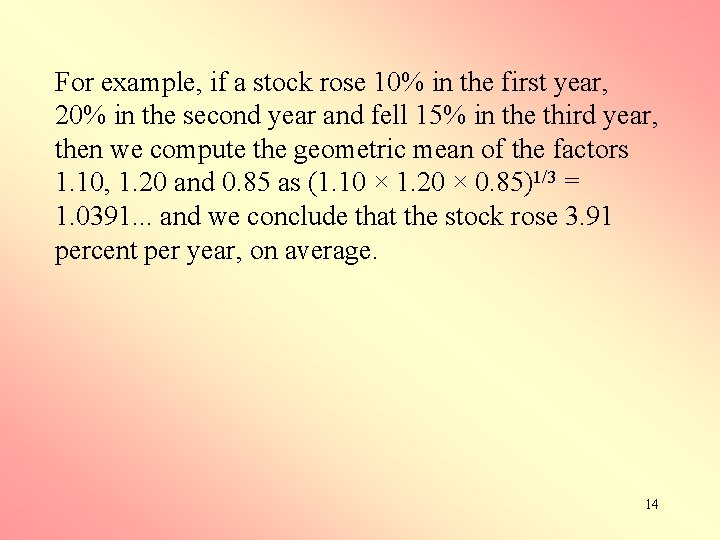For example, if a stock rose 10% in the first year, 20% in the second year and fell 15% in the third year, then we compute the geometric mean of the factors 1. 10, 1. 20 and 0. 85 as (1. 10 × 1. 20 × 0. 85)1/3 = 1. 0391. . . and we conclude that the stock rose 3. 91 percent per year, on average. 14Harmonic Mean is a "summary" statistic used in analyses of frequency data. The harmonic mean is sometimes used to average values that change in time. If a variable contains a zero (0) as a valid score, then the harmonic mean cannot be calculated (since it implies division by zero). 15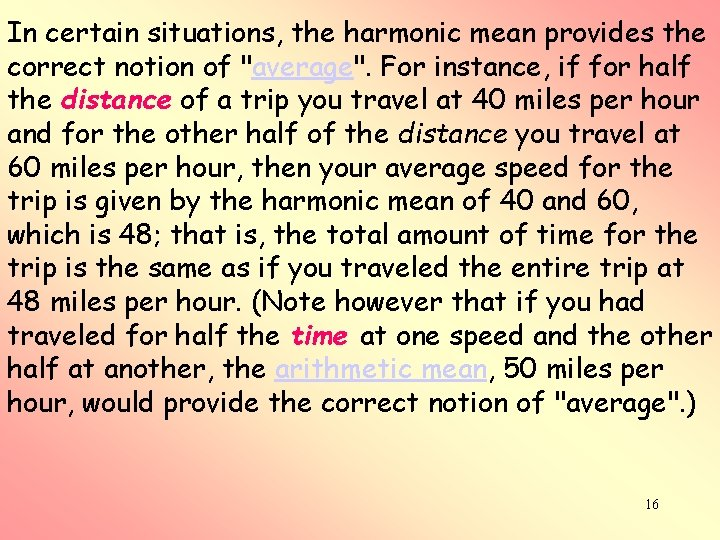In certain situations, the harmonic mean provides the correct notion of "average". For instance, if for half the distance of a trip you travel at 40 miles per hour and for the other half of the distance you travel at 60 miles per hour, then your average speed for the trip is given by the harmonic mean of 40 and 60, which is 48; that is, the total amount of time for the trip is the same as if you traveled the entire trip at 48 miles per hour. (Note however that if you had traveled for half the time at one speed and the other half at another, the arithmetic mean, 50 miles per hour, would provide the correct notion of "average". ) 16Proportion is a fraction in which the numerator is included within the denominator. A proportion is often expressed as a percentage. For example, we might describe the overall amount of smokers in a population as the proportion of people in the population who smoke. Suppose out of 125 people 15 smoke cigarette, then the proportion of people who smoke is P = 17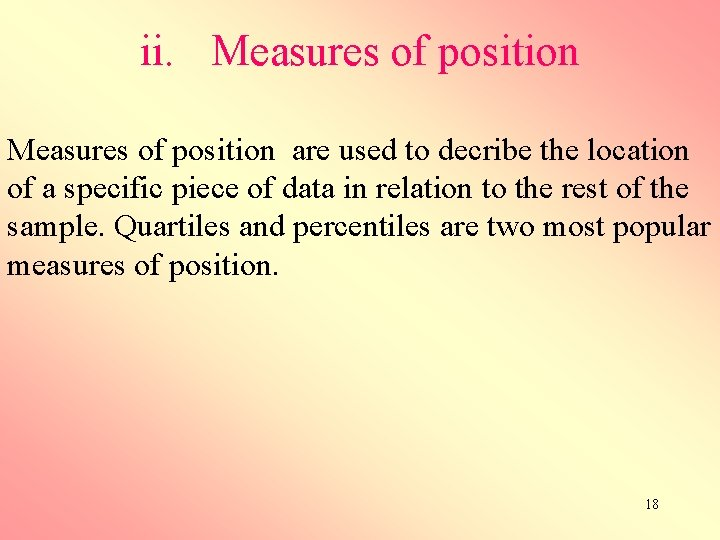ii. Measures of position are used to decribe the location of a specific piece of data in relation to the rest of the sample. Quartiles and percentiles are two most popular measures of position. 18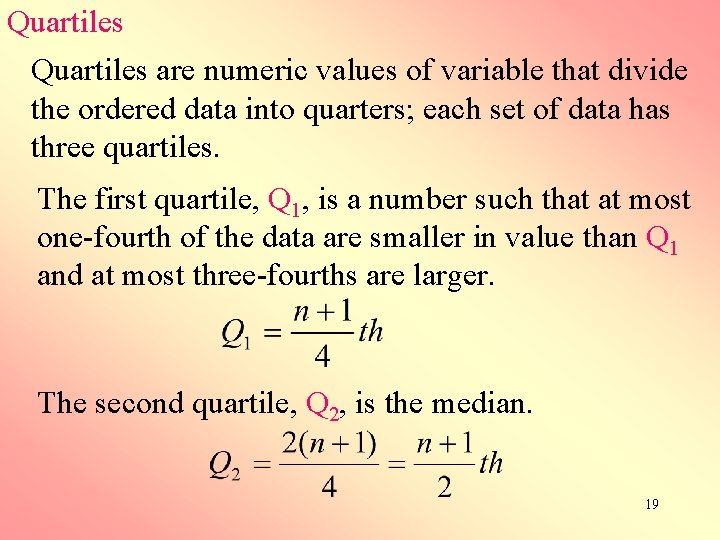Quartiles are numeric values of variable that divide the ordered data into quarters; each set of data has three quartiles. The first quartile, Q 1, is a number such that at most one-fourth of the data are smaller in value than Q 1 and at most three-fourths are larger. The second quartile, Q 2, is the median. 19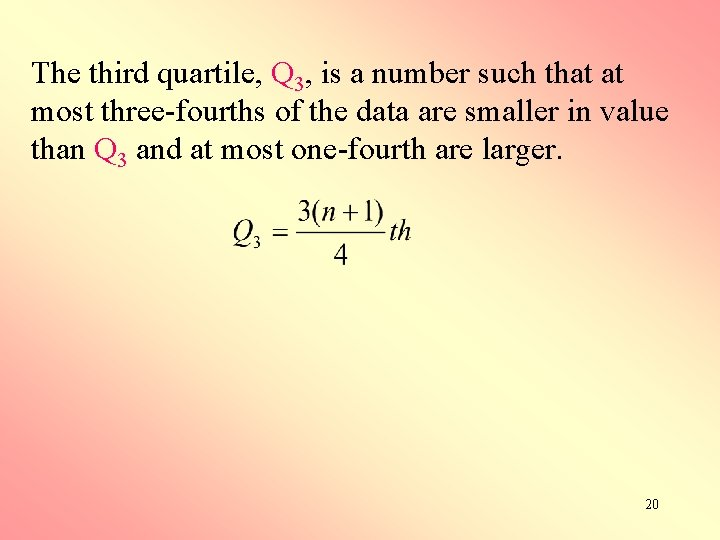The third quartile, Q 3, is a number such that at most three-fourths of the data are smaller in value than Q 3 and at most one-fourth are larger. 20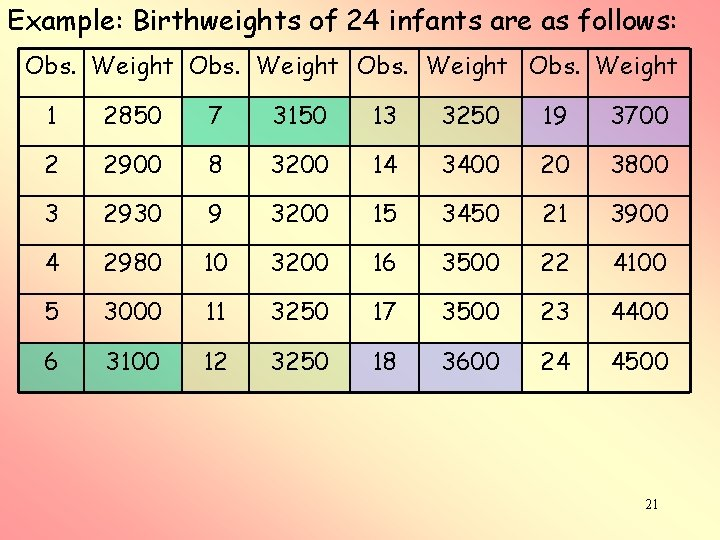Example: Birthweights of 24 infants are as follows: Obs. Weight 1 2850 7 3150 13 3250 19 3700 2 2900 8 3200 14 3400 20 3800 3 2930 9 3200 15 3450 21 3900 4 2980 10 3200 16 3500 22 4100 5 3000 11 3250 17 3500 23 4400 6 3100 12 3250 18 3600 24 4500 21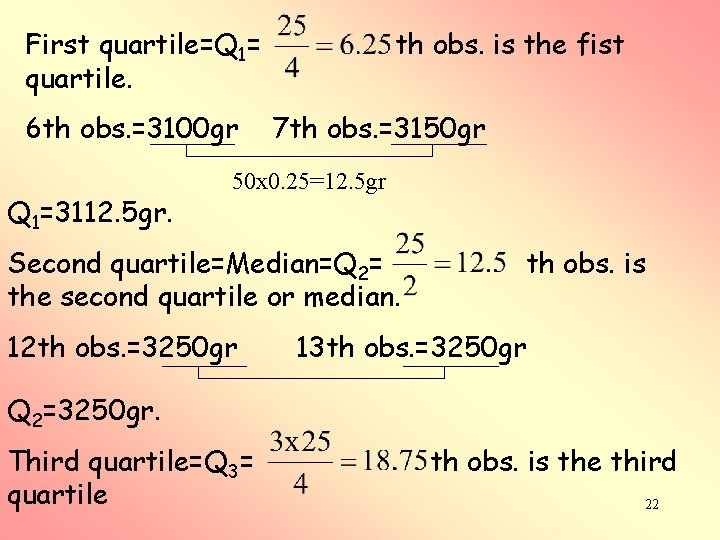First quartile=Q 1= quartile. 6 th obs. =3100 gr Q 1=3112. 5 gr. th obs. is the fist 7 th obs. =3150 gr 50 x 0. 25=12. 5 gr Second quartile=Median=Q 2= the second quartile or median. 12 th obs. =3250 gr th obs. is 13 th obs. =3250 gr Q 2=3250 gr. Third quartile=Q 3= quartile th obs. is the third 22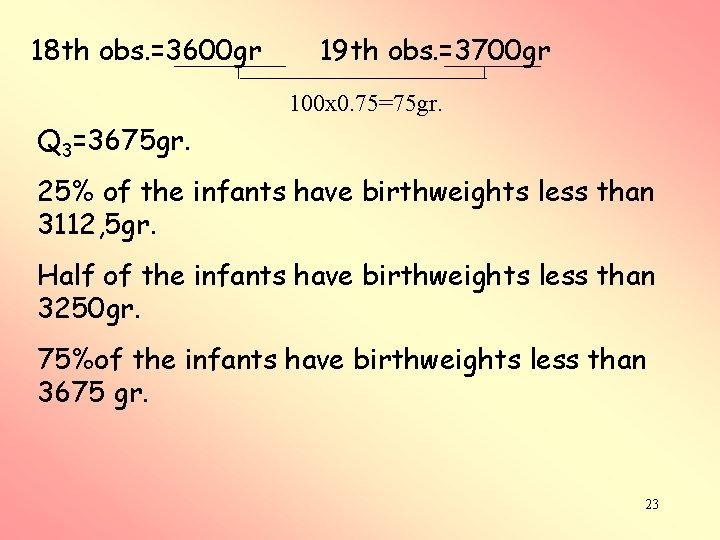18 th obs. =3600 gr 19 th obs. =3700 gr 100 x 0. 75=75 gr. Q 3=3675 gr. 25% of the infants have birthweights less than 3112, 5 gr. Half of the infants have birthweights less than 3250 gr. 75%of the infants have birthweights less than 3675 gr. 23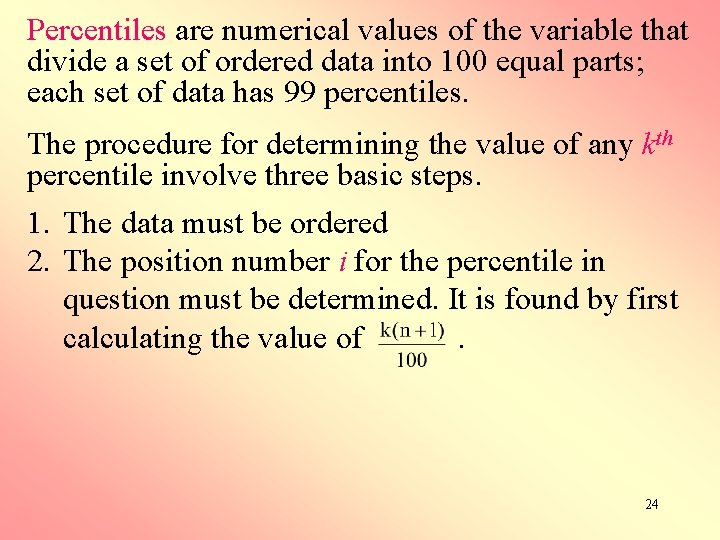Percentiles are numerical values of the variable that divide a set of ordered data into 100 equal parts; each set of data has 99 percentiles. The procedure for determining the value of any kth percentile involve three basic steps. 1. The data must be ordered 2. The position number i for the percentile in question must be determined. It is found by first calculating the value of . 24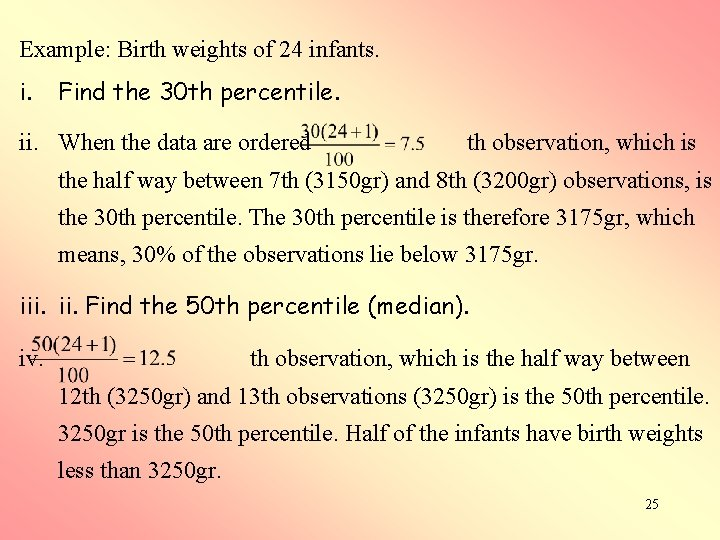Example: Birth weights of 24 infants. i. Find the 30 th percentile. ii. When the data are ordered th observation, which is the half way between 7 th (3150 gr) and 8 th (3200 gr) observations, is the 30 th percentile. The 30 th percentile is therefore 3175 gr, which means, 30% of the observations lie below 3175 gr. iii. Find the 50 th percentile (median). iv. th observation, which is the half way between 12 th (3250 gr) and 13 th observations (3250 gr) is the 50 th percentile. 3250 gr is the 50 th percentile. Half of the infants have birth weights less than 3250 gr. 252. Measures of dispersion Range The range is the simplest measure of dispersion. It is the difference between the highest valued (H) and the lowest valued (L) of the observations. Range= H-L 26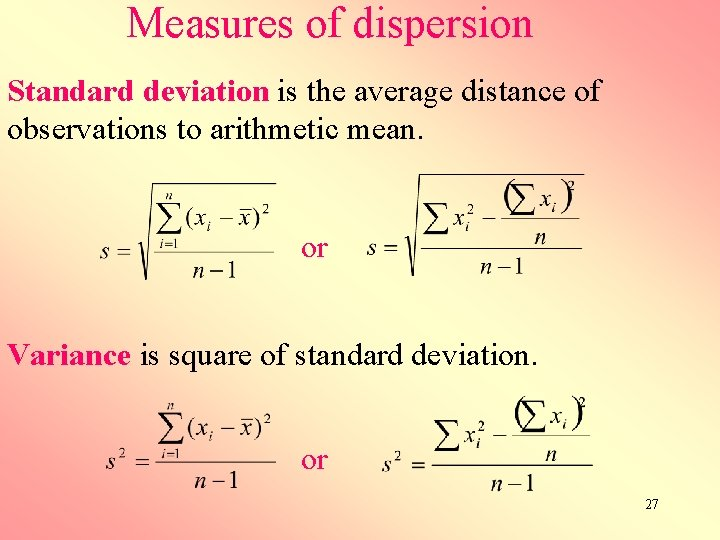Measures of dispersion Standard deviation is the average distance of observations to arithmetic mean. or Variance is square of standard deviation. or 27Step 1 Step 3 Step 4 Step 2 Step 5 NOTE: The sum of the deviation, , is always zero. 28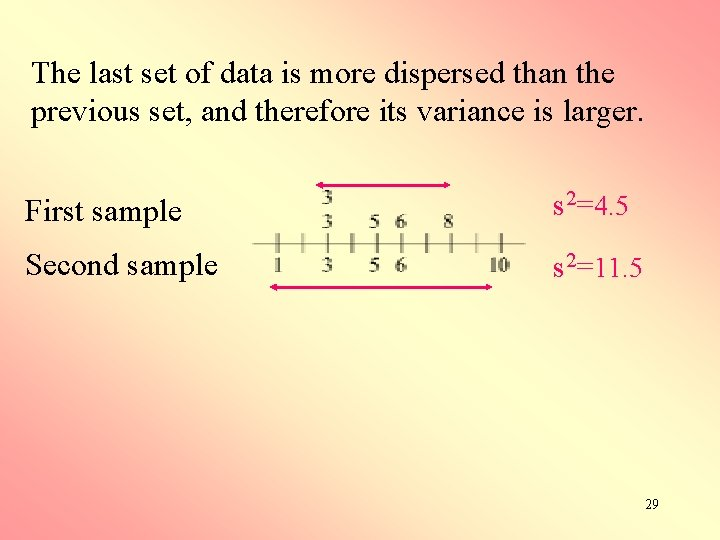The last set of data is more dispersed than the previous set, and therefore its variance is larger. First sample s 2=4. 5 Second sample s 2=11. 5 29Coefficient of variation (CV) shows the deviation as a percentage of arithmetic mean. 30Example: Heights of ten subjects are measured in cm and m. Data are listed below Although standard deviations are different, coefficient of variations are the same. s cv 31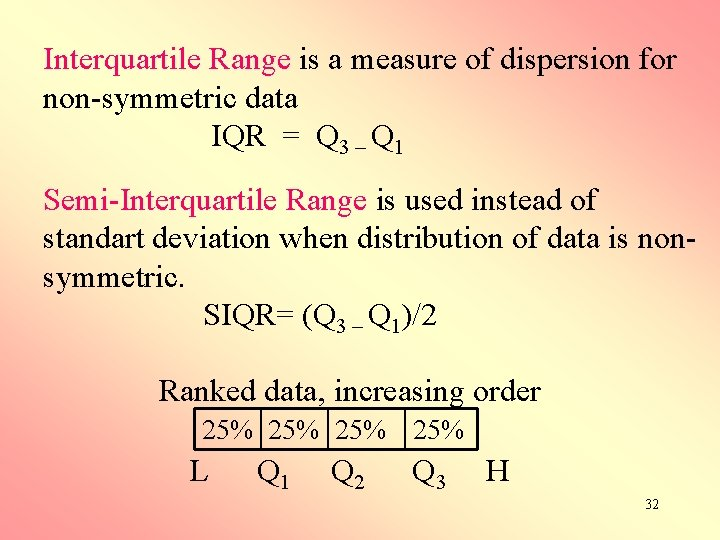Interquartile Range is a measure of dispersion for non-symmetric data IQR = Q 3 – Q 1 Semi-Interquartile Range is used instead of standart deviation when distribution of data is nonsymmetric. SIQR= (Q 3 – Q 1)/2 Ranked data, increasing order 25% 25% L Q 1 Q 2 Q 3 H 32